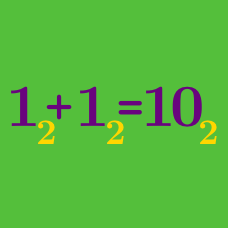Number Theory

# Arithmetic in Base N Warmup

$11 + 1 = 100$ In which base is this equation true?

$12 \times 3 = 40$ In which base is this equation true?

$\begin{array}{c c c} & \square & \bigtriangleup\\ + & \bigtriangleup & 8\\ \hline & A & 2 \end{array}$ If this calculation is written in base 12, which digit does $\square$ represent?

Note: In base 12, $A$ is the digit that represents the number written as 10 in base 10.

$\begin{array}{c c c c} & & 2 & 4\\ \times & & 1 & 4\\ \hline & & 9 & 1\\ & 2 & 4 & \\ \hline & 2 & D & 1 \end{array}$ Humans (typically) have 10 fingers, so we use base 10. The calculation above was done by an alien; how many fingers do these aliens have?

Note: Assume that the aliens use the same numeral conventions as humans; that is, their numerals (in order) are 0, 1, 2, ..., 8, 9, A, B, ...

$66 + 1 = 100$ In which base is this equation true?

×